Latest Banking jobs   »   Quantitative Aptitude Quiz For FCI Phase...

# Quantitative Aptitude Quiz For FCI Phase I 2023- 5th January

Q1. A shopkeeper sold two books each at price of Rs. 336. Out of two books one is sold at 12% profit and another at 16% loss. Find overall loss incurred in this process?
(a) Rs. 24
(b) Rs. 38
(c) Rs. 28
(d) RS. 32
(e) Rs. 36

Q2. From a group of 5 men and 4 women, five persons are to be selected to form a committee in which there should be at-least 3 men. In how many ways it can be done?
(a) 81
(b) 41
(c) 101
(d) 100
(e) 90

Q3. Mohit invested Rs. 3200 at 5% per annum compounded half yearly. Find amount earned as interest at the end of year?
(a) Rs. 122
(b) Rs. 162
(c) Rs. 81
(d) Rs. 102
(e) None of these.

Q4. Find semi-perimeter of semicircle which radius is 14cm?
(a) 72 cm
(b) 36 cm
(c) 18 cm
(d) 54 cm
(e) None of these.

Q5. Difference between 20% of x and 15% of y is 35 whereas difference between 30% of y and 15% of x is 30. Find (x + y)?
(a) 400
(b) 500
(c) 300
(d) 700
(e) 800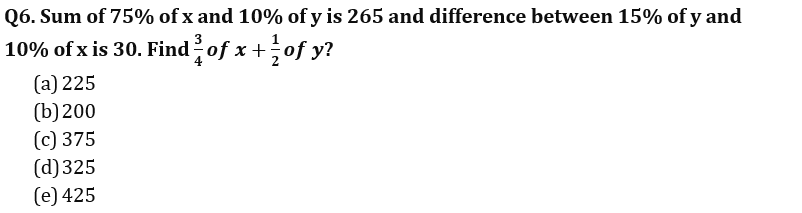Q7. Speed of a car is 30% more than speed of train. Train can cover one km in a minute, find in how much time car can cover 975 meters?
(a) 60 sec
(b) 30 sec
(c) 15 sec
(d) 45 sec
(e) 25 sec

Q8. Hemant marks up an article 40% above cost price and he gives 12% discount instead of 18% and earn Rs. 42 more, find mark price is how much more than cost price?
(a) Rs. 250
(b) Rs. 180
(c) Rs. 200
(d) Rs. 300
(e) Rs. 600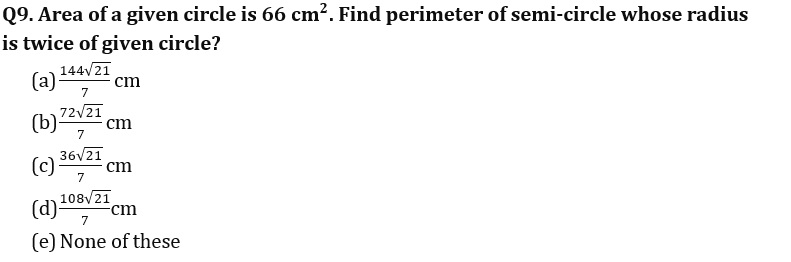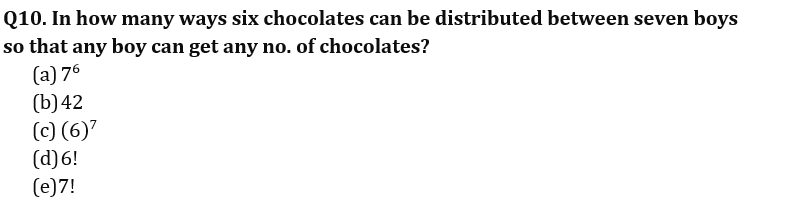Q11. Pipe A can fill a tank in 60 min and is 20% more efficient than B which is a filling pipe. There is pipe C which empties the tank. It takes 6/7 hours to fill the tank when all three opened together. Find in how much time pipe C alone can empty the tank?
(a) 60 min
(b) 120 min
(c) 110 min
(d) 75 min
(e) 90 min

Q12. A solid cylinder of height 12 cm and radius 5 cm is converted into few spherical solid balls of radius 1 cm. find how many balls are made.
(a) 225
(b) 275
(c) 350
(d) 325
(e) 175

Q13. A started a business with an investment of Rs 12000, after 6 months B joined him with Rs 15000 and after another 6 months C joined them with Rs 20000. If after end of two years profit share of B is Rs 9000, then what is the total profit earned by them?
(a) Rs 27000
(b) Rs 26400
(c) Rs 26600
(d) Rs 27760
(e) Rs 28860

Q14. A, B and C distribute a sum of money Rs 1250 such that C gets half of average of A and B share and B got 50% more than A. find share of B.
(a) Rs 400
(b) Rs 600
(c) Rs 250
(d) Rs 500
(e) Rs 300

Q15. In an examination, Dharam got 36% of maximum marks and failed by 48 marks, while Ankit got 46% of maximum marks which is 12 more than passing marks. Find the maximum marks.
(a) 500
(b) 750
(c) 600
(d) 450
(e) 650

Solutions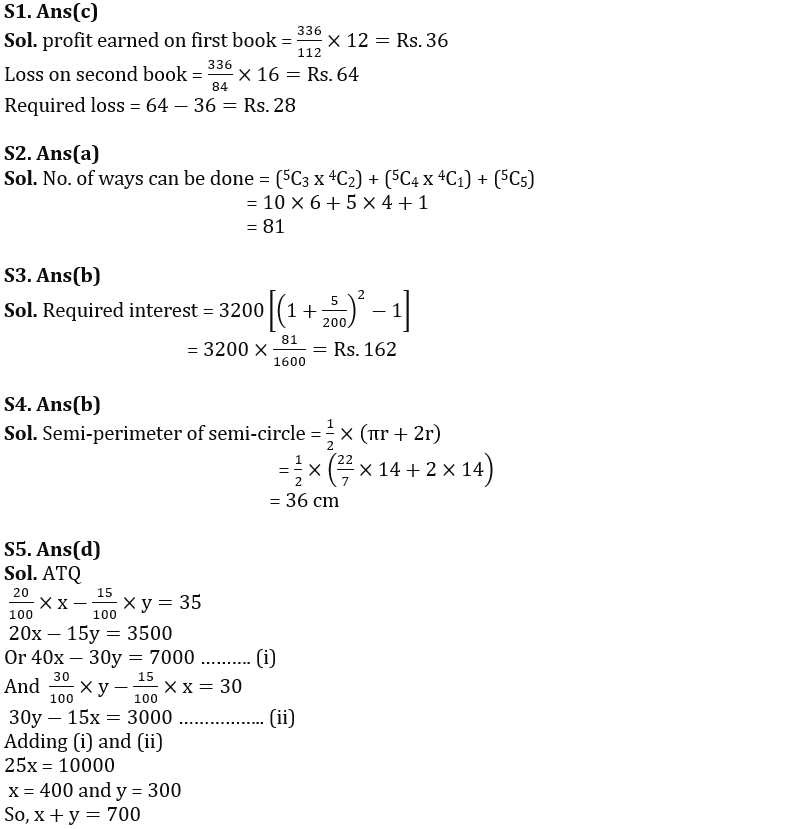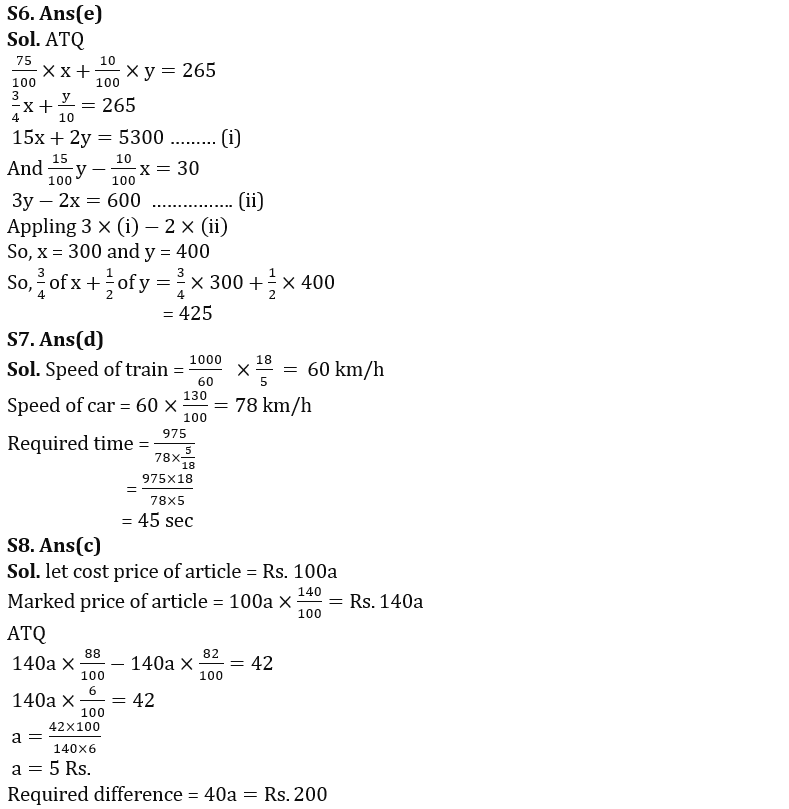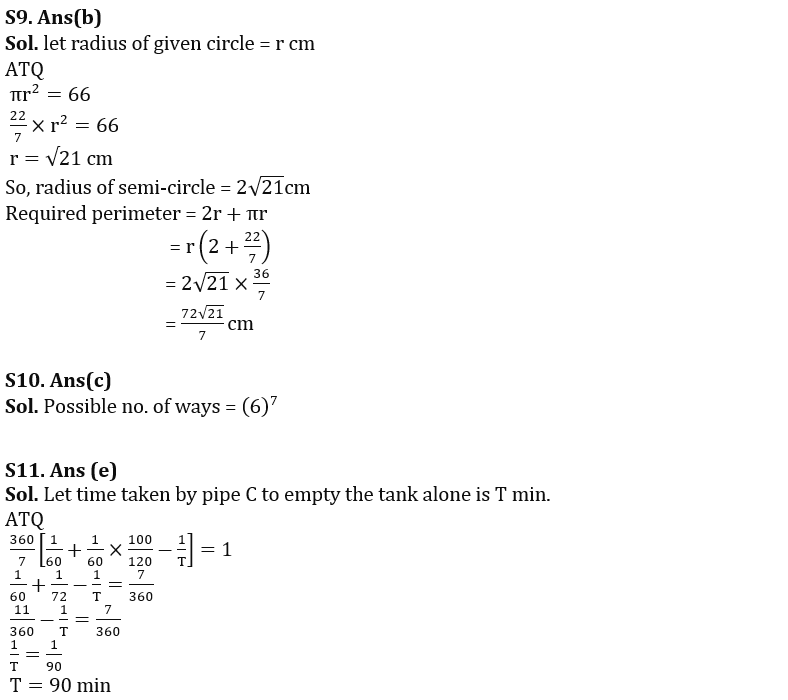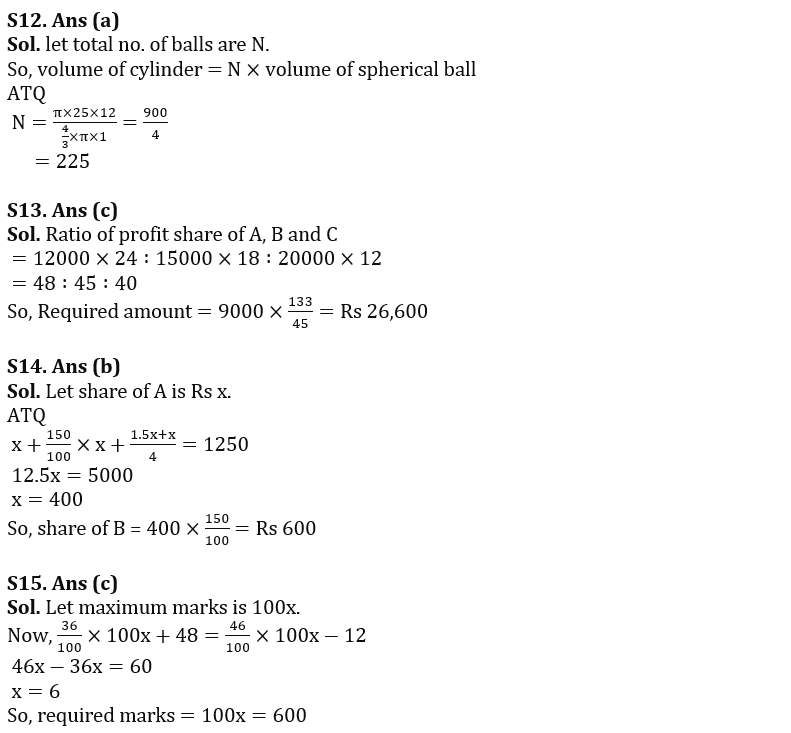## FAQs

#### Congratulations!